# Four families

Four families were on a joint trip. In the first family, there were three siblings, namely Alica, Betka and Cyril. In the second family were four siblings, namely David, Erik, Filip and Gabika. In the third family, there were two siblings, namely Hugo and Iveta. In the fourth family were three siblings, namely Ján, Karol and Lukáš. On the way, children were divided into groups so that in each group there were all children with the same number of brothers and no one else. How could children be divided? Specify all options.

Write to us the number of solutions ..

n =  2

### Step-by-step explanation: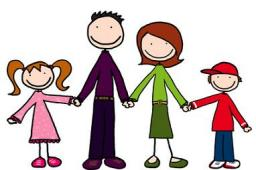Did you find an error or inaccuracy? Feel free to write us. Thank you!## Related math problems and questions:

• GroupA group of kids wanted to ride. When the children were divided into groups of 3 children, one remain. When divided into groups of 4 children, 1 remains. When divided into groups of 6 children, 1 remains. After divided into groups of 5 children, no one lef
• Lesson exercising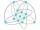The lesson of physical education, pupils are first divided into three groups so that each has the same number. The they redistributed, but into six groups. And again, it was the same number of children in each group. Finally they divided into nine equal g
• Two hundred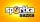Two hundred ten athletes competed in three athletics races on three fields. One hundred five athletes competed in the first, 60 in the second, and everyone else in the third. On the individual courts, the athletes were divided into groups. Each group, alt
• David numberJana and David train the addition of the decimal numbers so that each of them will write a single number and these two numbers then add up. The last example was 11.11. David's number had the same number of digits before the decimal point, the Jane's numbe
• On the tripOn the trip were more than 55 children, but less than 65 children. Groups of 7 could be formed, but groups of 8 no. How many children were on a trip?
• Families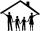In the class, we investigated how many children have families in which they live. How many % of pupils live in families with more than two children? If five families have one child in 9 families, have 2 children, in 5 families have 3 children, in 2 famili
• Number train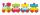The numbers 1,2,3,4,5,6,7,8 and 9 traveled by train. The train had three cars and each was carrying just three numbers. No. 1 rode in the first carriage, and in the last carriage was all odd numbers. The conductor calculated sum of the numbers in the firs
• Family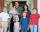A man and a woman have 6 daughters together (or - "with each-other" so that step-children are excluded). Each daughter has one brother. How many members are in the immediate family?
• GroupsIn the 6th class there are 60 girls and 72 boys. We want to divide them into groups so that the number of girls and boys is the same. How many groups can you create? How many girls will be in the group?
• MO Z6-6-1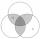Write integers greater than 1 to the blanks in the following figure so that each darker box was a product of the numbers in the neighboring lighter boxes. What number is in the middlebox?
• DecideThe rectangle is divided into seven fields. On each box is to write just one of the numbers 1, 2 and 3. Mirek argue that it can be done so that the sum of the two numbers written next to each other was always different. Zuzana (Susan) instead argue that i
• Group of childrenThere is a group of children. There is a boy named Adam in each of the three children subgroup and a girl named Beata in each quartet (four-member subgroup). How many children can be in such a group and what are their names in that case?
• Two groupsThe group of 10 girls should be divided into two groups with at least 4 girls in each group. How many ways can this be done?
• Square into three rectangles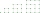Divide the square with a side length of 12 cm into three rectangles with have the same circumference so that these circumferences are as small as possible.
• Sports studentsThere are 120 athletes, 48 volleyball players, and 72 handball players at the school with extended sports training. Is it possible to divide sports students into groups so that the number in each group is the same and expressed by the largest possible num
• Squirrels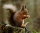The squirrels discovered a bush with hazelnuts. The first squirrel plucked one nut, the second squirrel two nuts, the third squirrel three nuts. Each new squirrel always tore one nut more than the previous squirrel. When they plucked all the nuts from the
• Clubhouse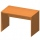There were only chairs and table in the clubhouse. Each chair had four legs, and the table was triple. Scouts came to the clubhouse. Everyone sat on their chair, two chairs were left unoccupied, and the number of legs in the room was 101. How many chairs